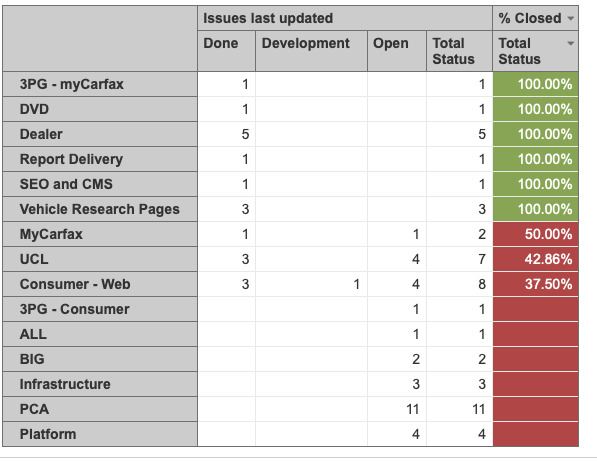# Show zero in calculated measure column

I have a report that calculates the percentage of work completed but I want to show zero where appropriate. I know there is a function CoalesceEmpty but can not figure out where to place in the custom field

CASE WHEN --represent only for Total Status and avoid dividing by zero
[Status].CurrentMember IS [Status].[Total Status] AND
([Measures].[Issues last updated],[Status].[Total Status]) <> 0

THEN --count if updated done issues divided by count of updated issues in total status
([Measures].[Issues last updated],[Status].[Done])
/
([Measures].[Issues last updated],[Status].[Total Status])
ENDYes, you are correct. You would like to use CoalesceEmpty function for this. I would suggest using it with a total of Done issues. Either there are some Done issues or there are 0 Done issues. Currently, a tuple for done issues will give you empty value and therefore there is empty value in the result as well.

Here is an updated formula with suggested change:

``````CASE WHEN --represent only for Total Status and avoid dividing by zero
[Status].CurrentMember IS [Status].[Total Status] AND
([Measures].[Issues last updated],[Status].[Total Status]) <> 0
THEN --count if updated done issues divided by count of updated issues in total status
CoalesceEmpty(([Measures].[Issues last updated],[Status].[Done]),0)
/
([Measures].[Issues last updated],[Status].[Total Status])
END
``````

Daina / support@eazybi.com